# Low-Light Face Recognition and Identity Verification Based on Image Enhancement

Low-Light Face Recognition and Identity Verification Based on Image Enhancement
Department of Informationization Construction and Management, Shaanxi University of Technology, Hanzhong 723000, China

Shaanxi Aviation Professional Technical Institute, Hanzhong 723102, China

Corresponding Author Email:
luqiuju@snut.edu.cn
Page:
513-519
|
DOI:
https://doi.org/10.18280/ts.390213
2 December 2021
|
Accepted:
25 February 2022
|
Published:
30 April 2022
| Citation

OPEN ACCESS

Abstract:

After years of development, face recognition is now a relatively perfect technology. It is non-contact, intuitive, simple, accurate, and applicable to complex practical environments. To a certain extent, the application of deep learning has enhanced the accuracy of face recognition. But there are some defects with deep learning in detecting face objects of different types in different environments, calling for further explorations. Therefore, this paper explores the low-light face recognition and identity verification based on image enhancement. Specifically, light processing and Gaussian filtering were adopted to suppress and eliminate the low-light effect of low-light face images. The basic framework and objective function of the existing generative adversarial network (GAN) were modified. By learning the mapping of side and front faces in multi-pose face images in the image space, a cross-pose GAN was established to turn faces of different poses into front faces. The proposed model was proved effective through experiments.

Keywords:

low-light image, face recognition, image enhancement, identity verification

1. Introduction

The efficient management of crowdy places like governments, enterprises, and schools hangs on the efficient, fast, and safe verification and certification of the identities of the personnel entering and leaving these places [1-7]. Biometric personnel identification verifies the identity of a person, using his/her unique measurable or identifiable physical and behavioral features [8-17]. These physical features include face, fingerprint, palm print, retina, iris, and DNA, and the behavioral features include signature, voice, gait, etc. Among them, face recognition has evolved into a relatively perfect technology. It is non-contact, intuitive, simple, accurate, and applicable to complex practical environments. The application of deep learning to face recognition has enhanced the robustness, widened the applicable scope, elevated the accuracy, and guaranteed the security of face verification [18-22].

Sridhar et al.  compared two low-rank matrix approximation algorithms based on robust principal component analysis (RPCA) with the other algorithms in terms of the low-rank recovery performance on occluded face images, and proposed a new method that recognizes individuals in any given image, using the data histogram of the recovered sparse image. Deep learning algorithms based on convolutional neural network (CNN) are widely applied in computer vision. Zhu et al.  proposed a deep CNN (DCNN)-based low-light face recognition method, which enhances the low-light face image with multiscale Retinex, and imports the processed signals into a four-layer DCNN. The classification model was generated through iterative training of the neural network. Many state-of-the-art face recognition models cannot effectively detect the faces in images captured in low light. This problem can be solved by enhancing the illumination of the face images before face recognition. Huang and Chen  evaluated the relevant enhancement methods, and put forward a new feature reconstruction network based on these findings. A feature map was generated from the original face image and the enhanced image, without changing the illumination of face features. Focusing on low-light face images, Yang et al.  proposed an adaptive algorithm based on Otsu’s segmentation algorithm to collect images adaptively under low illumination. The AdaBoost classifier was adopted to verify the low-light face images before and after processing. Their model successfully enhances the accuracy of face detection. Mudunuri and Biswas  proposed a fully automatic approach to identify low-resolution face images captured in an uncontrolled environment: Multidimensional scaling was adopted to learn a common transform matrix for the entire face, which simultaneously transforms the face features of low- and high-resolution training images, such that the distance between the two images is close to that between the two images captured under same controlled imaging conditions. Their approach was tested on challenging real-world databases, and compared with the most advanced strategy, a super-resolution, classifier-based, cross-modal synthesis technique. The results demonstrate the effectiveness of their approach.

The face images collected in complex public environments are constrained by multiple factors, including pixels, size, and light balance. These constraints limit the detection accuracy of face recognition methods. The application of deep learning improves the accuracy of face recognition to a certain extent. But there are some defects with deep learning in detecting face objects of different types in different environments, calling for further explorations. Therefore, this paper explores the low-light face recognition and identity verification based on image enhancement. Section 2 adopts light processing and Gaussian filtering to suppress and eliminate the low-light effect of low-light face images. Section 3 modifies the basic framework and objective function of the existing generative adversarial network (GAN), and establishes a cross-pose GAN to turn faces of different poses into front faces, by learning the mapping of side and front faces in multi-pose face images in the image space. The proposed model was proved effective through experiments.

2. Low-Light Face Image Enhancement

To realize the intelligent monitoring of crowdy places, biometric identity verification is more convenient and accurate than traditional identity verification strategies. It is more suitable for practical scenarios like security and anti-terrorism. Figure 1 provides an example of identity verification. If the application scenario requires the identity verification to be invisible or applicable to non-cooperative personnel, face recognition would be the favored choice.

The low-light face recognition aims to recognize face images with low illumination, complex background, and low quality. In a low-light face image, there are not much recognizable information. It is difficult to extract complete face features for sample matching. To minimize the effect of illumination on final face recognition rate, the low-light effect of the low-light face image must be suppressed and eliminated.

## 1.png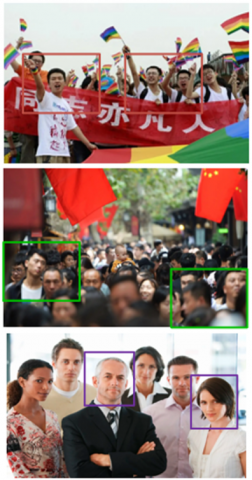Figure 1. Example of identity verification

2.1 Light processing

In this paper, the light of the low-light face image is enhanced through the histogram equalization, a technique of illumination normalization. A low-light color face image can be illustrated by the red-green-blue (RGB) color space. Here, the component of each channel, red, green, or blue, is equalized separately, and the three resulting components are combined as the output. Let g(n,m) be the input low-light face image; h(a,b) be the output image; R be the set of coordinates including the neighborhood center (a,b); N be the number of pixels in the neighborhood. Then, the histogram equalization can be expressed as:

$h\left( a,b \right)=\frac{1}{N}\sum\nolimits_{\left( n,m \right)\in R}{g\left( n,m \right)}$     (1)

2.2 Gaussian filtering

In a low-light face image, any pixel containing the noise induced by the lack of illumination is much darker than the other pixels in the neighborhood. If the other pixels are ranked in descending order, the bottom pixels must be noisy, while the middle pixels are not likely to have noise. Therefore, this paper adopts the median filter to process the noisy pixels in the low-light face image. Let a1,a2 ......am be m pixels in the low-light face image, which can be sorted in ascending order by pixel value as ai1<ai2<... ... aim. Then, the median can be calculated by:

b=\left\{ \begin{align} & {{a}_{{{i}_{\left( \frac{m+1}{2} \right)}}}},m\text{ }is\text{ }an\text{ }odd\text{ }number \\ & \frac{1}{2}\left[ {{a}_{{{i}_{\left( \frac{m}{2} \right)}}}}+{{a}_{{{i}_{\left( \frac{m+2}{2} \right)}}}} \right],m\text{ }is\text{ }an\text{ }even\text{ }number \\\end{align} \right.      (2)

3. Multi-Pose Face Recognition

In the unrestricted scene of low-light face recognition, there are few image samples for model training. The features of these samples are distributed unevenly. Adding to the difficulty of feature extraction in face recognition, the performance of deep learning-based face recognition depends on the type of the recognition model, and the uncertainty and other factors of face changes in the collected face images. After collecting multi-pose face images, the face recognition is generally achieved by face pose correction based on two-dimensional (2D) or three-dimensional (3D) template. But oversampling may occur due to the small key feature area of the face.

The human face changes in three dimensions: pitch angle, yaw angle, and roll angle. In collected images, the variation of the yaw angle often affects face recognition. To solve the problem, this paper modifies the basic framework and objective function of the existing GAN, and establishes a cross-pose GAN to turn faces of different poses into front faces, by learning the mapping of side and front faces in multi-pose face images in the image space.

Each collected multi-pose face image carries a label of the identity of the personnel in the image. After processing, the image will have real textures, and a front view. The original and processed images with the same identity label were treated as two training targets of the proposed GAN model.

To achieve the training goal, and to make the discriminator always judge the output image as true, the generator, which receives multiple multi-pose face images, must maximize the adversarial loss. Let TXO be the multi-pose face image. The encoder HBM will extract the deep eigenvector of the input TXO. The decoder HYM reconstructs the extracted deep eigenvector into a face image with front view: TXR=HYM(HBM(TXO)). Therefore, the objective function for generator optimization consists of two parts: the adversarial loss and the correlation loss. The former loss substantiates the adversarial training with the discriminator, while the latter loss represents the corrected front face features. Let L be the size of a small batch; TXOi be the i-th multi-pose face image being inputted. The adversarial loss can be expressed as:

$SU_{CL}^{H}=\frac{1}{L}\sum\limits_{i=1}^{L}{\log C\left( H\left( TX_{i}^{O} \right) \right)}$    (3)

For each collected multi-pose face image, the symmetry between the pixels in the left and right faces is compared. Let Q and F be the width and height of the corrected front face image, respectively; TXr and TXg be the generated front face and the true front face, respectively. Then, the symmetric loss can be expressed as:

$S{{U}_{SL}}=\frac{1}{Q/2\times F}\sum\limits_{i=1}^{Q/2}{\sum\limits_{j=1}^{F}{\left| TX_{i,j}^{r}-TX_{Q-\left( i-1 \right),j\quad}^{r} \right|}}$     (4)

There is no absolute relationship between the pixels corresponding to TXr and those corresponding to TXg. However, the pixel changes in the corresponding key areas in multi-pose face image are continuous. To ensure consistency in key areas, eyes, nose, and mouth are denoted by k=1,2,3; the size of a key area is denoted by ||ok||; the pixel coordinates in a key area are denoted by (i,j). Then, the objective function can be expressed as:

$S{{U}_{IU}}=\frac{1}{3}\sum\limits_{k=1}^{3}{\frac{1}{\left\| {{o}^{k}} \right\|}}\sum\limits_{i}{\sum\limits_{j}{\left| TX_{o_{i,j}^{k}}^{r}-TX_{o_{i,j}^{k}}^{g} \right|}}$      (5)

Under the constraint of the above objective function, the generator reconstructs the input multi-pose face images into the desired face image with a front view.

## 2.pngFigure 2. Dimensional matching of face recognition on multi-pose face images

In the actual application of face recognition and identity verification, the face image acquisition device usually collects a series of face images with continuously changing features. The face recognition of multi-pose face images can be significantly improved, if the string of images can be deeply fused to realize the complementarily between face features. The rotation information of the face can be represented by a 3D rotation vector. This paper converts the rotation vector into a rotation matrix through the Rodrigues transform. The Euler angles of faces with different poses are thus acquired to generate the face offset angle. Figure 2 shows the dimensional matching of face recognition on multi-pose face images.

For rotation vector ŝ=(sa,sb,sc), the modulus length is denoted by φ, and the unit vector is represented by ŝ←ŝ/φ. Then, the rotation matrix can be expressed as:

\begin{align} & S=cos\phi *TX+\left( 1-cos\phi \right)*\hat{s}{{{\hat{s}}}^{T}} \\ & +sin\phi \left[ \begin{matrix} 0 & -{{s}_{c}} & {{e}_{b}} \\ {{s}_{c}} & 0 & -{{s}_{c}} \\ -{{s}_{b}} & {{s}_{c}} & 0 \\\end{matrix} \right] \\\end{align}      (6)

The face poses of the multi-pose face image can be decomposed into three different rotation angles: pitch angle, yaw angle, and roll angle, which are rotated around the a-axis, the b-axis, and the c-axis, respectively. If the rotation matrix can be expressed as:

$S=\left[ \begin{matrix} {{s}_{11}} & {{s}_{12}} & {{s}_{13}} \\ {{s}_{21}} & {{s}_{22}} & {{s}_{23}} \\ {{s}_{31}} & {{s}_{32}} & {{s}_{33}} \\\end{matrix} \right]$     (7)

According to the C-A-B turning system, the conversion from S to each Euler angle can be completed by:

${{\phi }_{c}}=xtan2\left( {{s}_{32}},{{s}_{33}} \right)$     (8)

${{\phi }_{b}}=xtan2\left( -{{s}_{31}},\sqrt{s_{11}^{2}+s_{21}^{2}} \right)$      (9)

${{\phi }_{c}}=xtan2\left( {{s}_{21}},{{s}_{11}} \right)$      (10)

Let o be the radian of the yaw angle for the obtained multi-pose face image; $\theta \in$[0, 1] be the confidence coefficient required for compression. If the face in the multi-pose face image looks at the front, then θ=1, and the face contributes the most to the complementarity of face features. As θ gradually decreases from 1 to 0, the face turns from the front to the side. In this case, the face contributes less and less to the complementarity of face features. The compression from o to θ satisfies:

$\theta ={{e}^{-{{o}^{2}}}}$       (11)

Thus, the eigenvector supporting the complementarity of face features is the weighted average of the eigenvector of each image in the collected series of multi-pose face images:

\begin{align} & g\left( {{H}_{enc}}\left( TX_{1}^{O} \right),{{H}_{enc}}\left( TX_{2}^{O} \right),...,{{H}_{enc}}\left( TX_{n}^{O} \right) \right) \\ & =\sum\limits_{i=1}^{n}{{{\theta }_{i}}}{{H}_{enc}}\left( TX_{i}^{O} \right)/\sum\limits_{i=1}^{n}{{{\theta }_{i}}} \\\end{align}       (12)

## 3.png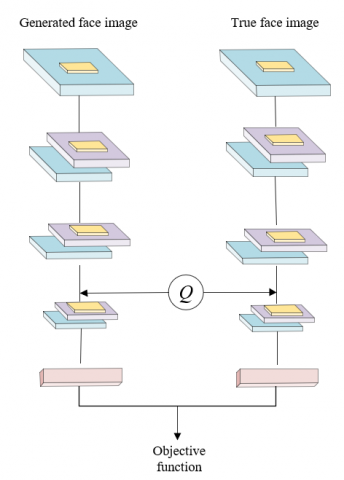Figure 3. Structure and workflow of the discriminator

Based on the series of multi-pose face images, the front face can be generated as:

$T{{X}^{r}}={{H}_{dec}}\left( \sum\limits_{i=1}^{n}{{{\theta }_{i}}}{{H}_{enc}}\left( TX_{i}^{O} \right)/\sum\limits_{i=1}^{n}{{{\theta }_{i}}} \right)$     (13)

This paper classifies the series of multi-pose face images, with the aid of the discriminator in the cross-pose GAN. Two inputs TXr and TXg were designed for the discriminator based on the multi-task CNN (MTCNN). The discriminator can judge whether a collected multi-pose face image is true or false, and recognize the identity of the personnel in the input face images. The output of the discriminator is an M+1-dimensional vector, where the first N dimensions are the eigenvector of the input face image, and the last 1 dimension is used to judge the true or false state of the image. Figure 3 shows the structure and workflow of the discriminator.

The loss function for discriminator optimization can be expressed as:

$mi{{n}_{C}}S{{U}_{C}}=-SU_{CL}^{C}+\beta S{{U}_{AN}}$      (14)

Let CM+1 be the M+1-th element outputted by the discriminator, which is responsible to judge whether the input multi-pose face image is true. Then, the adversarial loss in the above formula can be calculated by:

\begin{align} & SU_{CL}^{C}={{T}_{T{{X}^{o}}\tilde{\ }o\left( TX \right)}}\left[ \log {{C}^{M+1}}\left( T{{X}^{O}} \right) \right] \\ & +{{T}_{T{{X}^{r}}\tilde{\ }{{o}_{r}}\left( TX \right)}}\left[ \log \left( 1-{{C}^{M+1}}\left( T{{X}^{r}} \right) \right) \right] \\\end{align}      (15)

The eigenvector distance SUAN between two input images must be minimized, such that the generated front face has the same identity label with the true front face. Let CM be the first M-dimensional eigenvector outputted by the discriminator. Then, the minimization can be expressed as:

$S{{U}_{AN}}=\left\| {{C}^{M}}\left( T{{X}^{r}} \right)-{{C}^{M}}\left( T{{X}^{g}} \right) \right\|_{2}^{2}$      (16)

Through the above training, the proposed discriminator can judge the true or false state of the inputted series of multi-pose face images, while acquiring the deep eigenvectors of faces. The obtained deep eigenvectors are then classified by the classifier. The results can be directly applied to verify the identity of personnel.

4. Experiments and Results Analysis

It is not necessarily good to have more complementary face features. Figure 4 compares the face recognition accuracy at different number of complementary face features. A series of continuous and non-overlapping face images were collected, and imported to our network model. With the increase of complementary face features, the face recognition accuracy first increased and the decreased. On average, face recognition accuracy reached the best level, when there were four face features.

In addition, four commonly used classical databases were selected. Each face image was divided, such that the local features are evenly distributed in each zone. Under this premise, the image resolution was reduced by 2-8 times to test the face recognition rate. Figure 5 shows the face recognition rates at different resolutions.

## 4.pngFigure 4. Relationship between the number of complementary face features and the recognition accuracy

## 5.pngFigure 5. Face recognition rates at different resolutions

As shown in Figure 5, the face recognition rate decreased gradually with the decline of image resolution on each dataset. When the resolution dropped by 4 or more times, the recognition rate on each dataset fell below 60%, failing to meet the actual needs of face recognition. Thus, the original resolution was reduced by 4 times, and the resulting images were taken as the targets of simulation.

Figure 6 displays the effect of block level and quantization level on the accuracy of low-light face recognition. Theoretically, the description degree of the feature information of the face image is determined by the fineness of the block and quantization. If the block level and the quantization level are high, it will lead to feature redundancy, increase the computational complexity, and reduce the computational speed. If the block level and quantization level are too small, the generated face features will lack statistical significance. As shown in Figure 6, the face recognition rate was high, when the resolution was 502 or 602, and the face was divided into 8 local feature zones; the face recognition rate was optimal, when the resolution was 302 or 402, the face was divided into 10 local feature zones.

Figure 7 demonstrates the effect number of training samples and resolution on recognition accuracy of low-level face images. The proposed face feature complementation algorithm was applied to classify and recognize five different face poses. As shown in Figure 7, the recognition accuracy of each type of pose was above 80%, when the resolution was 502, and the training set size was 400. The recognition accuracy of each type of pose was above 84%, when the resolution was 602, and the training set size was 450. In addition, the faces were recognized more and more accurately, with the growing number of training samples. Thus, the face recognition and identity verification of low-light images need a sufficiently large training set. However, the recognition effect on images containing strongly left-skewed or right-skewed faces was not satisfactory, even if there were lots of samples.

To test the robustness of our algorithm to illumination, the samples with normal illumination in the sample set were treated as training samples, and the other samples as test samples. Figure 8 shows the influence of illumination on low-light face recognition accuracy. As shown in Figure 8, our algorithm recognized faces more accurately than principal component analysis (PCA), MTCNN, and DCNN. Therefore, the proposed low-level face recognition algorithm, which is based on face feature complementation, can extract light-invariant features from face images excellently, as long as the illumination changes in the required range.

## 61.png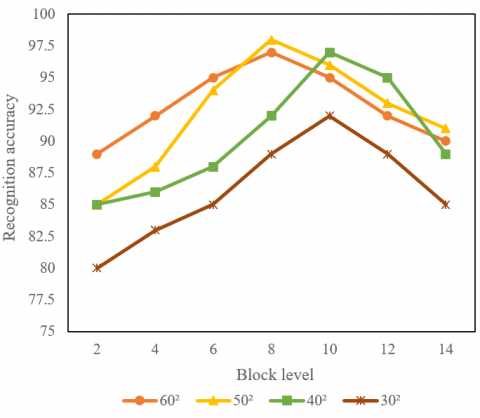(1) Block level

## 62.png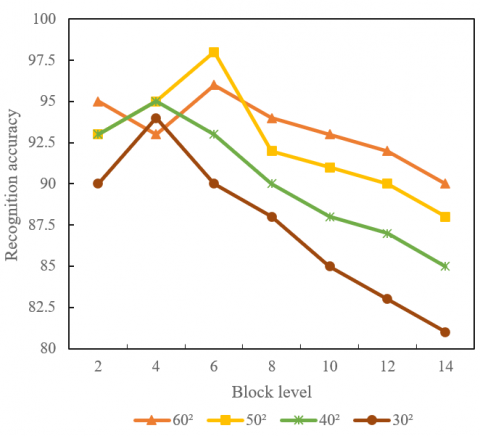(2) Quantization level

Figure 6. Effect of block level and quantization level on low-light face recognition accuracy

## 71.png(1) Number of training samples

## 72.png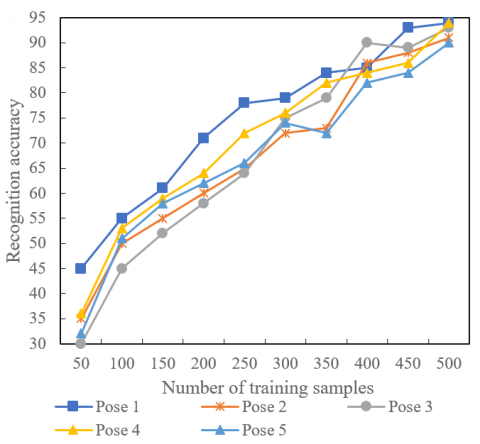(2) Resolution

Figure 7. Effect of number of training samples and resolution on recognition accuracy of low-level face images

## 8.png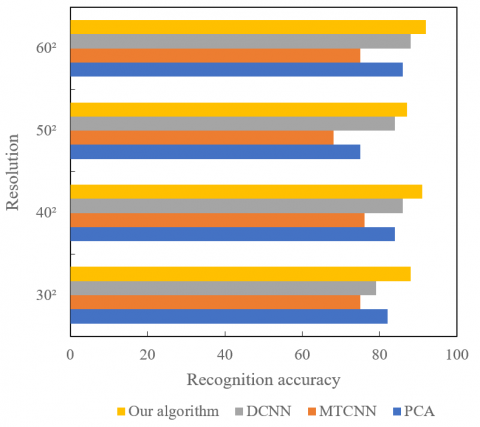Figure 8. Effect of illumination on recognition accuracy of low-level face images

5. Conclusions

Based on image enhancement, this paper explores the low-level face recognition and identity verification. Firstly, the low-light effect of low-light face images was suppressed and eliminated through light processing and Gaussian filtering. Next, the basic framework and objective function of the existing GAN were revised, and a cross-pose GAN was established to transform faces of different poses into front faces. The transform was realized by learning the mapping of side and front faces in multi-pose face images in the image space. In addition, the authors analyzed the relationship between the number of complementary face features and the recognition accuracy, revealing that, on average, face recognition accuracy reached the best level, when there were four face features. Moreover, the authors investigated how the recognition accuracy of low-level face images is influenced by block level and quantization level, by the number of training samples and resolution, and by the illumination. The results show that the proposed low-level face recognition algorithm, which is based on face feature complementation, can extract light-invariant features from face images excellently, as long as the illumination changes in the required range.

References

 Shi, C., Du, M., Lu, W., He, X., Lu, S. (2022). Identity authentication with association behavior sequence in machine-to-machine mobile terminals. Mobile Networks and Applications, 27(1): 96-108. https://doi.org/10.1007/s11036-020-01706-0

 Prajapati, V., Gupta, B.B. (2022). A robust authentication system with application anonymity in multiple identity smart cards. Journal of Information Technology Research (JITR), 15(1): 1-21. https://doi.org/10.4018/JITR.2022010107

 Gupta, B.B., Gaurav, A., Chui, K.T., Hsu, C.H. (2022). Identity-based authentication technique for IoT devices. In 2022 IEEE International Conference on Consumer Electronics (ICCE), pp. 1-4. https://doi.org/10.1109/ICCE53296.2022.9730173

 Said, W., Mostafa, E., Hassan, M.M., Mostafa, A.M. (2022). A multi-factor authentication-based framework for identity management in cloud applications. CMC-Computers Materials & Continua, 71(2): 3193-3209.

 Hossain, M.J., Xu, C., Li, C., Mahmud, S.H., Zhang, X., Li, W. (2021). ICAS: Two-factor identity-concealed authentication scheme for remote-servers. Journal of Systems Architecture, 117: 102077. https://doi.org/10.1016/j.sysarc.2021.102077

 Yang, Q., Wang, C., Wang, C., Teng, H., Jiang, C. (2020). Fundamental limits of data utility: A case study for data-driven identity authentication. IEEE Transactions on Computational Social Systems, 8(2): 398-409. https://doi.org/10.1109/TCSS.2020.3035462

 Song, X., Liu, Y., Deng, H., Xiao, Y. (2018). High-dimensional quantum threshold anonymous identity authentication scheme. Quantum Information Processing, 17(9): 1-17. https://doi.org/10.1007/s11128-018-1969-8

 Fietkau, J., Seifert, J.P. (2018). Swipe your fingerprints! how biometric authentication simplifies payment, access and identity fraud. In 12th USENIX Workshop on Offensive Technologies (WOOT 18).

 Zhu, H., Zhang, Y., Wang, X. (2016). A novel one-time identity-password authenticated scheme based on biometrics for e-coupon system. Int. J. Netw. Secur., 18(3): 401-409.

 Zhang, X., Xu, C., Zhang, Y. (2017). Fuzzy identity-based signature scheme from lattice and its application in biometric authentication. KSII Transactions on Internet and Information Systems (TIIS), 11(5): 2762-2777. https://doi.org/10.3837/tiis.2017.05.025

 Ghosh, S., Majumder, A., Goswami, J., Kumar, A., Mohanty, S.P., Bhattacharyya, B.K. (2016). Swing-pay: One card meets all user payment and identity needs: A digital card module using NFC and biometric authentication for peer-to-peer payment. IEEE Consumer Electronics Magazine, 6(1): 82-93. https://doi.org/10.1109/MCE.2016.2614522

 Zhu, H., Xia, Y., Li, H. (2015). An efficient and secure biometrics-based one-time identity-password authenticated scheme for e-coupon system towards mobile Internet. Journal of Information Hiding and Multimedia Signal Processing, 6(3): 444-457.

 Ouannes, L., Ben Khalifa, A., Essoukri Ben Amara, N. (2021). Comparative study based on de-occlusion and reconstruction of face images in degraded conditions. Traitement du Signal, 38(3): 573-585. https://doi.org/10.18280/ts.380305

 Tommandru, S., Sandanam, D. (2020). An automated framework for patient identification and verification using deep learning. Revue d'Intelligence Artificielle, 34(6): 709-719. https://doi.org/10.18280/ria.340605

 Gedik, O., Demirhan, A. (2021). Comparison of the effectiveness of deep learning methods for face mask detection. Traitement du Signal, 38(4): 947-953. https://doi.org/10.18280/ts.380404

 Stefanova, M., Stefanov, S., Asenov, O. (2012). Identity protection accessing e-government through the biometric authentication methods. In 2012 6th IEEE International Conference Intelligent Systems, pp. 403-408. https://doi.org/10.1109/IS.2012.6335250

 Cheng, H., Rong, C., Tan, Z., Zeng, Q. (2012). Identity based encryption and biometric authentication scheme for secure data access in cloud computing. Chinese Journal of Electronics, 21(2): 254-259.

 Kowalski, M., Grudzień, A. (2018). High-resolution thermal face dataset for face and expression recognition. Metrology and Measurement Systems, 25(2): 403-415. https://doi.org/10.24425/119566

 Wen, G., Chen, H., Cai, D., He, X. (2018). Improving face recognition with domain adaptation. Neurocomputing, 287: 45-51. https://doi.org/10.1016/j.neucom.2018.01.079

 Patil, S.A., Deore, P.J. (2018). High dimensional video-based face recognition. International Journal of Circuits, Systems and Signal Processing, 12: 674-683.

 Gao, J., Xu, M., Wang, H., Zhou, J. (2022). End-to-end saliency face detection and recognition. Journal of Physics: Conference Series, 2171(1): 012004. https://doi.org/10.1088/1742-6596/2171/1/012004

 Elharrouss, O., Almaadeed, N., Al-Maadeed, S., Khelifi, F. (2022). Pose-invariant face recognition with multitask cascade networks. Neural Computing and Applications, 1-14. https://doi.org/10.1007/s00521-021-06690-4

 Sridhar, K.V., Chandravathi, C., Raghuvamshi, H., Kumar, S. (2021). Performance analysis of weighted low rank model with sparse image histograms for face recognition under lowlevel illumination and occlusion. In 2021 International Conference on Intelligent Technologies (CONIT), pp. 1-10. https://doi.org/10.1109/CONIT51480.2021.9498351

 Zhu, Y., Ni, X., Wang, H., Yao, Y. (2020). Face recognition under low illumination based on convolutional neural network. International Journal of Autonomous and Adaptive Communications Systems, 13(3): 260-272.

 Huang, Y.H., Chen, H.H. (2020). Face recognition under low illumination via deep feature reconstruction network. In 2020 IEEE International Conference on Image Processing (ICIP), pp. 2161-2165. https://doi.org/10.1109/ICIP40778.2020.9191321

 Yang, A., Wang, Q., Cao, J. (2019). Research on adaptive face recognition algorithm under low illumination condition. In Advances in Graphic Communication, Printing and Packaging, 266-272. https://doi.org/10.1007/978-981-13-3663-8_37

 Mudunuri, S.P., Biswas, S. (2015). Low resolution face recognition across variations in pose and illumination. IEEE Transactions on Pattern Analysis and Machine Intelligence, 38(5): 1034-1040. https://doi.org/10.1109/TPAMI.2015.2469282